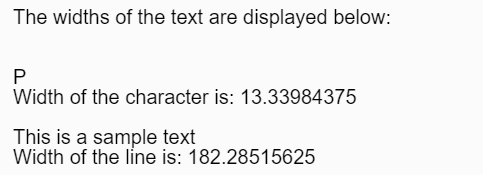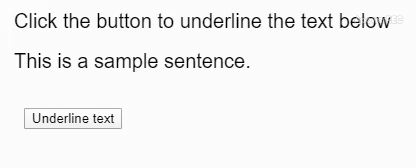GeeksforGeeks App
Open AppBrowser
Continue

## Related Articles

The textWidth() function is used to calculate the width of the text given as parameter. Syntax:

`textWidth( theText )`

Parameters: This function accepts a single parameter as mentioned above and described below:

• theText: It holds the string of which the width has to be measured.

Return Value: It returns a number which denotes the width of the given text. Below examples illustrate the textWidth() function in p5.js: Example 1:

## javascript

 `let sampleChar = "P";``let sampleLine = "This is a sample text";`` ` `// Canvas area creating``function` `setup() {``  ``createCanvas(400, 200);``  ``textSize(20);``  ``text(``'The widths of the text are '``        ``+ ``'displayed below:'``, 20, 20);``   ` `  ``// Checking textwidth sampleChar``  ``text(sampleChar, 20, 80);``  ``let charWidth = textWidth(sampleChar);``  ``text("Width of the character is: "``        ``+ charWidth, 20, 100);``   ` `  ``// Checking textwidth sampleLine``  ``text(sampleLine, 20, 140);``  ``let lineWidth = textWidth(sampleLine);``  ``text("Width of the line is: "``        ``+ lineWidth, 20, 160);``}`

Output:Example 2: Using text width measure to underline the text.

## javascript

 `let sampleText =``    ``"This is a sample sentence.";`` ` `// Canvas area creating``function` `setup() {``  ``createCanvas(400, 200);``  ``textSize(20);``  ``text(``'Click the button to underline'``        ``+ ``' the text below'``, 20, 40);``  ``text(sampleText, 20, 80);``   ` `  ``// Creating button``  ``btn = createButton("Underline text");``  ``btn.position(30, 120);``  ``btn.mousePressed(underlineText);``}`` ` `// Measuring text width and``// creating underline``function` `underlineText() {``  ``let width = textWidth(sampleText);``  ``line(20, 90, width + 20, 90);``}`

Output:Online editor: https://editor.p5js.org/ Environment Setup: https://www.geeksforgeeks.org/p5-js-soundfile-object-installation-and-methods/ Reference: https://p5js.org/reference/#/p5/textWidth

My Personal Notes arrow_drop_up
Related Tutorials